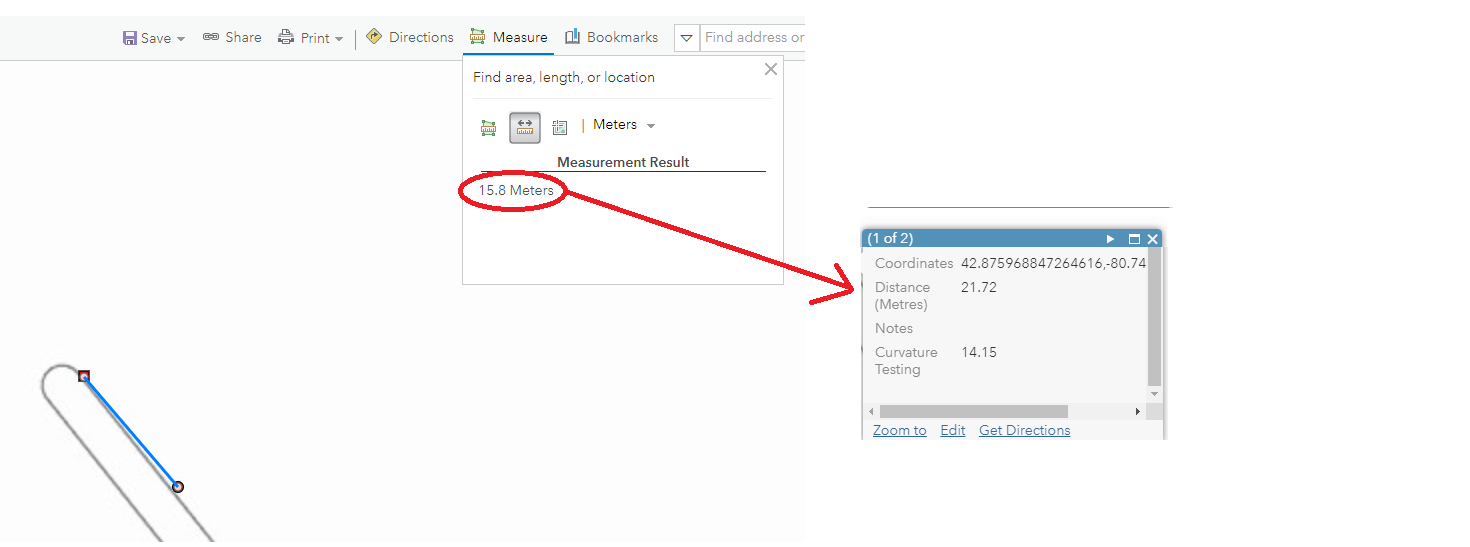208
0
03-06-2019 09:02 AMNew Contributor III

The Distance function gives a planar distance. However, I would like the distance accounting for the earth's curvature / coordinate system.. where it is similar to the measuring tool in AGOL.
Is there another way in arcade to make an expression calculate the distance accounting for the coordinate system & earth's curvature so that it is very similar to the measuring tool in ArcGIS Online?I've tried a function (Curvature Testing) like this:
algorithm - Calculate distance between two latitude-longitude points? (Haversine formula) - Stack Ov...
and it is close to the measuring tool but not quite.

Tags (3)
0 Replies